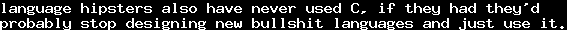# The Glivenko TheoremThu, 23 Jun 2011 03:00:00 GMT

As the German Wikipedia keeps getting destroyed by arrogant pimple faced pubescent high schoolers, unlike the English Wikipedia, there is no article about the Glivenko Theorem in the German Wikipedia anymore. As far as I remember, there has been one in the past. Currently, this article in the English Wikipedia is suggested to be merged with the article about the Gödel Gentzen Negative Translation, which sounds reasonable, as it is a generalization - but it also does not have an article in the German Wikipedia anymore.

The German Wikipedia does not have any form of the quality it used to have. There is no "freedom" anymore: The last time I edited something, it took weeks (!) for the article's usurper to confirm my changes. I do not see the "freedom" it proclaims anymore.

Besides general boredness, I take that as an occasion to blog about the Glivenko Theorem. Especially, I want to try out how good (or bad) I can get MathJax to produce proof trees. Apparently, it does not look so bad, but there is no real possibility to create horizontallines, stuff like \cline does not work in MathJax. If someone has suggestions on how to make them look better, please let me know. I am using my own pre-generated HTML tables currently, which look good, but I would prefer a solution that is more integrated into MathJax.

Especially, this post's primary purpose is to be informative for people who were not concerned with logic so far. Every mathematician should already know everything stated here.

The Glivenko Theorem sais that in propositional logic, if P→Q is provable classically, then ¬¬P→¬¬Q is provable intuitionistically.

There are several ways to prove this. But let us first look at what that means.

In the end, a sufficiently simple calculus for this is the Hilbert Calculus, which only implements Modus Ponens, that is, has only one rule: From A and A→B we may derive B. Additionally, if we have a proof of B from A, we may derive A→B and strike A from the list of axioms.

 A→B A B

 A ⋮ B A→B

We get intuitionistic propositional logic by the axiom schemes (→ is right-associative and binds less strict than ∨ and ∧)

A→A∨B, B→A∨B, A→B→A∧B

A∨B→(A→C)→(B→C)→C, A∧B→(A→B→C)→C

Furthermore, we introduce a special propositional symbol ⊥, the falsum, and add the axiom of ex falso quodlibet

⊥→A

which means that from a wrong proposition we may derive everything.

For classical logic, we define ¬A:=A→⊥, "not A". Then to get classical logic, we add the axiom scheme of duplex negatio affirmat

((A→⊥)→⊥)→A

which becomes

¬¬A→A

in the above notation.

To prove the Glivenko Theorem, we show that every rule and axiom for classical logic is derivable in double-negated form in intuitionistic logic. Therefore, the first thing we shall prove is that from ¬¬(A→B) and ¬¬A we may derive ¬¬B, corresponding to the modus ponens. In words, the proof goes:

Assuming A→B and ¬B, we know that ¬A must also hold, which contradicts ¬¬A. Therefore we know that one of our assumptions is wrong. Assuming ¬(A→B), we get a contradiction with ¬¬(A→B). Therefore, the assumption ¬B must be wrong, therefore ¬¬B.

Formally, it goes:

 [A→B] [A] [¬B] B ⊥ ¬¬A ¬A ⊥ ¬¬(A→B) ¬(A→B) ⊥ ¬¬B

Furthermore, we need to show that if ¬¬B can be implied by ¬¬A, also ¬¬(A→B) holds. So let us assume that ¬¬B follows from ¬¬A, but ¬(A→B). Assuming ¬A holds, using ex falso quodlibet ⊥→B gives us A→B which contradicts ¬(A→B). So ¬¬A which implies - by assumption - ¬¬B. Now, assuming B, we can follow A→B, which also contradicts ¬(A→B), so ¬B. But ¬¬B and ¬B contradict each other. So ¬¬(A→B). I admit, this proof is somewhat hard to understand. Here is the formal tree:

 [¬A] [A] ⊥→B ⊥ B ¬(A→B) A→B ⊥ [B] ¬¬A [¬(A→B)] A→B ⋮ ⊥ ¬¬B ¬B ⊥ ¬¬(A→B)

Notice that from B we followed A→B. This is correct according to the above definitions, but maybe not clear immediately.

An easier proof can be given for A→¬¬A, which we need to be able to convert all of our additional axioms for the junctors.

 A [¬A] ⊥ ¬¬A

Finally, we need ¬¬(¬¬A→A) to be derivable, and by the above proof, it is sufficient to prove ¬¬¬¬A→¬¬A, because that is ¬¬(¬¬A)→¬¬(A) and therefore implies ¬¬((¬¬A)→(A)). It can be derived by:

 [¬¬A] [¬A] ⊥ ¬¬¬¬A ¬¬¬A ⊥ ¬¬A

We have proved the Glivenko Theorem. W00t.